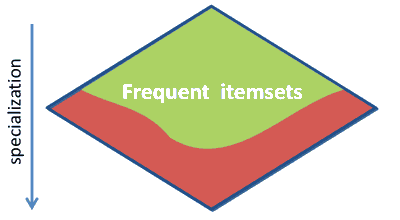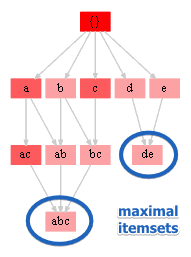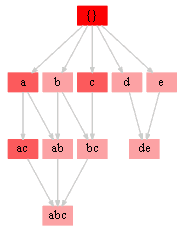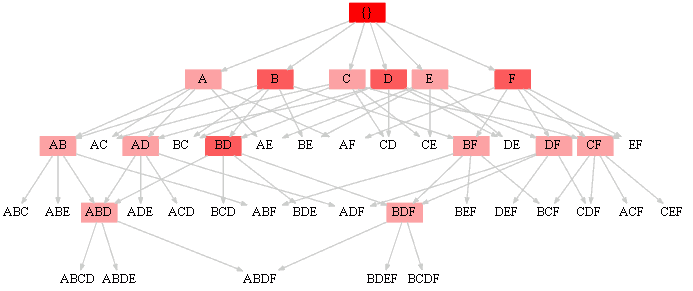ML Wiki

Apriori

This is an algorithm for Frequent Pattern Mining based on Breadth-First Search traversal of the itemset Lattice

Downward Closure

This method uses the property of this Lattice:

•• $X \subseteq Y, \text{supp}(Y) = t \Rightarrow \text{supp}(X) \geqslant t$
• $X \supseteq Y, \text{supp}(X) < \text{min_t} \Rightarrow \text{supp}(Y) < \text{min_t}$

Idea

• at a step $k$
• generate phase
• generate potentially frequent interesting itemsets of size $k$ based on the previous step
• test phase
• scan the generated itemsets
• eliminate non-frequent ones

Algorithm

Apriori(dataset $D$, frequency threshold $t$)

• $C_1 = \{ \text{all itemsets of len 1} \}$
• $i \leftarrow 1$
• while $C_i \not \equiv \varnothing$
• $F_i \leftarrow \{ c \in C_i | \text{supp}(c, D) \geqslant t \}$
• $C_{i+1} \leftarrow \{ \text{generate ($i+1$)-itemsets having$i+1$frequent itemsets} \}$
• $i \leftarrow i+1$
• return $F_0 \ \cup \ ... \ \cup \ F_i$

Python

def frequency(D, itemset):
return sum([1 for T in D if itemset.issubset(T)])

def s(S, i): return frozenset(S | {i})

I = 'abcdef'
D = [set(x) for x in ['abc', 'acdef', 'abc', 'de']]

th = 2
frequent_items = []

C = [frozenset([i]) for i in I]

while C:
F = [i for i in C if frequency(D, i) >= th]
C = {s(T, i)  for i in I   for T in F   if i not in T}
frequent_items.extend(F)

res = [''.join(sorted(T)) for T in frequent_items]

Maximal Frequent Itemsets

There's no need to store all the frequent itemsets

• we can store only the maximal ones (ones that that have no superset)
• and generate the rest from them

Consider the result obtained by the algorithm:

• $\{a, b, c, d, e, bc, ac, ab, de, abc\}$
•• there are two maximal sets: \text{de} and \text{abc}
• so, if we generate all subsets of these two, we'll get all the frequent itemsets
>>> powerset('de') | powerset('abc')
# sorted([''.join(i) for i in powerset('de') | powerset('abc')],
#        key=lambda x: (len(x), x))
['', 'a', 'b', 'c', 'd', 'e', 'ab', 'ac', 'bc', 'de', 'abc']

Examples

Example 1

• items ${a,b,c,d,e,f}$
• threshold $t = 3$
• 4 transactions
• $\{ abc, acdef, abc, de \}$
• notation: $\{ X \to \text{frequency}(X) \}$

step 0

• $C_1 \leftarrow \{a \to 3, b \to 2, c \to 3, d \to 2, e \to 2, f \to 1\}$

step 1

• $F_1 \leftarrow \{a \to 3, b \to 2, c \to 3, d \to 2, e \to 2\}$
• $C_2 \leftarrow \{ce \to 1, df \to 1, bf \to 0, af \to 1, ae \to 1, ad \to 1, bd \to 0, ef \to 1, be \to 0, bc \to 2, ac \to 3, cd \to 1, cf \to 1, ab \to 2, de \to 2\}$

step 2

• $F_2 \leftarrow \{bc \to 2, ac \to 3, ab \to 2, de \to 2\}$
• $C_3 \leftarrow \{abc \to 2, abe \to 0, ade \to 1, bde \to 0, bcd \to 0, bcf \to 0, bce \to 0, ace \to 1, acd \to 1, abd \to 0, def \to 1, cde \to 1, abf \to 0, acf \to 1\}$

step 3

• $F_3 \leftarrow \{abc \to 2\}$
• $C_4 \leftarrow \{abcd \to 0, abce \to 0, abcf \to 0\}$

step 4

• $F_4 \leftarrow \{\}$
• $C_5 \leftarrow \{\}$

final result:

• $\{a, b, c, d, e, bc, ac, ab, de, abc\}$
•• note that for $abc$ to be selected, all $ab$, $ac$, $bc$ have to be frequent itemsets

Example 2

${A, B, C, D, E, F}$

• $T_1 = \{A,B,D,E\}$
• $T_2 = \{A,B,C,D,F\}$
• $T_3 = \{B,D,F\}$
• $T_4 = \{C,E,F\}$

Run:

• step 0
• $C: [(A, 2), (B, 3), (C, 2), (D, 3), (E, 2), (F, 3)]$
• step 1
• $F: [(A, 2), (B, 3), (C, 2), (D, 3), (E, 2), (F, 3)]$
• $C: [(CE, 1), (DF, 2), (BF, 2), (AF, 1), (AE, 1), (AD, 2), (BD, 3),$
• $(BE, 1), (BC, 1), (AC, 1), (CD, 1), (EF, 1), (DE, 1), (AB, 2), (CF, 2)]$

step 2

• $F: [(DF, 2), (BF, 2), (AD, 2), (BD, 3), (AB, 2), (CF, 2)]$
• $C: [(ABC, 1), (ABE, 1), (CDF, 1), (ACF, 1), (BCF, 1), (BCD, 1),$
• $(BDF, 2), (ADF, 1), (ACD, 1), (BEF, 0), (ABD, 2), (BDE, 1),$
• $(CEF, 1), (DEF, 0), (ABF, 1), (ADE, 1)]$
• step 3
• $F: [(BDF, 2), (ABD, 2)]$
• $C: [(ABCD, 1), (BDEF, 0), (BCDF, 1), (ABDF, 1), (ABDE, 1)]$
• step 4
• $F: []$
• final result: $[A, B, C, D, E, F, DF, BF, AD, BD, AB, CF, BDF, ABD]$
•Note the step 2:

• we have $BD$ and $DF$. to take $BDF$ we also need to have $DF$.
• do we have it? yes $\Rightarrow$ take it
• Even though we have $AB$ and $BF$, there's no $AF$
• so not taking $ABF$

Remark:

• Maximal frequent itemsets are $E, CF, ABD$ and $BDF$.
• $X$ is maximal $\iff$ there $\not \exists \ Y, Y \supset X : \text{freq}(Y) > \text{freq}(X)$
• any frequent itemset is included in one of the maximal ones
• this is useful for checking if a pattern frequent or not:
• if it's not contained in any of the maximal frequent sets, it's not frequent